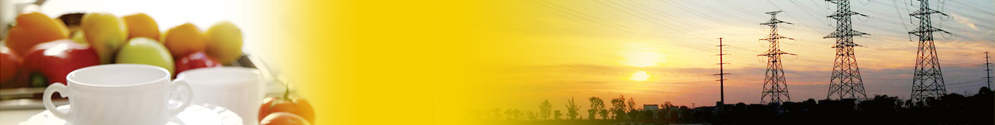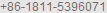www.finnedtubes.cn | Site map | Contact | Download Catalogue | Print | Favorite | 中文magvant.cnBoiler tubes Heat exchanger tubes Welded pipes L, LL, KLM finned tubes (fin tubes) high frequency welded (serrated) finned tubes (fin tubes) (serrated) extruded finned tubes hot dipped galvanized oval (elliptical) finned tubes, rectangular fin tubes condenser fin flat tubes H / double H finned tubes (fin tubes) Studded Pipes Laser welded finned tubes (fin tubes) G embedded finned tubes (fin tubes) inner finned tubes (fin tubes) longitudinal finned tubes (fin tubes) seamless finned tubes with two longitudinal opposite fins casting alloy tubes Tube finning machine Aluminum plates, strips,foils Aluminum welding wire rod Aluminum tubes, profile Economizer for Boiler air-cooled heat exchanger (air cooler) material standard & specification

ASTM A333 steel tubes

ASTM A333 / A333M - 11 Standard Specification for Seamless and Welded Steel Pipe for Low-Temperature Service

This specification covers wall seamless and welded carbon and alloy steel pipe intended for use at low temperatures. The pipe shall be made by the seamless or welding process with the addition of no filler metal in the welding operation. All seamless and welded pipes shall be treated to control their microstructure. Tensile tests, impact tests, hydrostatic tests, and nondestructive electric tests shall be made in accordance to specified requirements.

Referenced Documents (purchase separately) The documents listed below are referenced within the subject standard but are not provided as part of the standard.

ASTM Standards

A370 Test Methods and Definitions for Mechanical Testing of Steel Products
A671 Specification for Electric-Fusion-Welded Steel Pipe for Atmospheric and Lower Temperatures
A999/A999M Specification for General Requirements for Alloy and Stainless Steel Pipe
E23 Test Methods for Notched Bar Impact Testing of Metallic Materials
E165 Practice for Liquid Penetrant Examination for General Industry
E709 Guide for Magnetic Particle Testing

ASME Boiler and Pressure Vessel Code

 standard material tensile(MPa) yield(MPa) E(%) temperature | - ? ? ASTM A333 Grade 1 =380 =205 =35 =25 -50 -45 Grade 3 =450 =240 =30 =20 -150 -100 Grade 4 =415 =240 =30 =16.5 -150 -100 Grade 6 =415 =240 =30 =16.5 -50 -45 Grade 7 =450 =240 =30 =22 -100 -75 Grade 8 =690 =515 =22 -320 -195 Grade 9 =435 =315 =28 -100 -75 Grade 10 =550 =450 =22 -75 -60 Grade 11 =450 =240 =18

Chemical compositions of ASTM 333 steel grades (%)

 standard material C Si Mn P S Cr Ni ASTM A333 Grade 1 =0.30 0.40-1.06 =0.025 =0.025 Grade 3 =0.19 0.18-0.37 0.31-0.64 =0.025 =0.025 3.18-3.82 Grade 4 =0.12 0.18-0.37 0.50-1.05 =0.025 =0.025 0.44-1.01 0.47-0.98 Cu0.40-0.75, Mo0.04-0.30 Grade 6 =0.30 =0.10 0.29-1.06 =0.025 =0.025 Grade 7 =0.19 0.13-0.32 =0.90 =0.025 =0.025 2.03-2.57 Grade 8 =0.13 0.13-0.32 =0.90 =0.025 =0.025 8.40-9.60 Grade 9 =0.20 0.40-1.06 =0.025 =0.025 1.60-2.24 Cu0.75-1.25 Grade 10 =0.20 0.10-0.35 1.15-1.50 =0.03 =0.015 =0.15 =0.25 Cu=0.015, Mo=0.50, V=0.12, Al=0.06 Grade 11 =0.10 =0.35 =0.6 =0.025 =0.025 =0.50 35.0-37.0 Mo=0.50

Search >>
 web magvant.com

 Contact Us >> Phone:Fax: +86-510-68930106 E-mail: info@magvant.com Technical communication tech@magvant.com www.magvant.com
 Terms & conditions Privacy statement